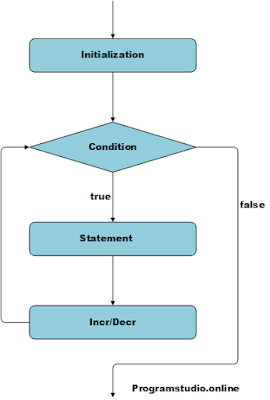### C++ For Loop

The C++ for loop is employed to iterate a neighborhood of the program several times. If the amount of iteration is fixed, it's recommended to use for loop than while or do-while loops.

The C++ for loop is same as C/C#. we will initialize variable, check condition and increment/decrement value.

A for loop may be a repetition control structure that permits you to efficiently write a loop that must execute a selected number of times.

Flow Chart: -C++ For Loop

C++ For Loop

Syntax: -

for (initialize;condition; incr/decr){
//Code are going to be executed
}

Example: -

#include <iostream>
using namespace std;

int main() {
for (int i=1;i <= 5; i++){
cout << i <<endl;
}
return 0;
}

When the above code is compiled and executed, it produces the following Output −

Output:
1
2
3
4
5
C++ Nested For Loop

In C++, we will use for loop inside another for loop, it's referred to as nested for loop. The inner loop is executed fully when outer loop is executed just one occasion . So if outer loop and inner loop are executed 4 times, inner loop are going to be executed 4 times for every outer loop

Syntax: -

for (initialize;condition; incr/decr){
for (initialize;condition; incr/decr){
//Code are going to be executed
}
}

Example: -

#include <iostream>
using namespace std;

int main() {
for (int i=1;i <= 2; i++)
{
for (int j=1;j <= 2; j++)
{
cout << i <<" "<< j <<endl;
}
}
return 0;
}

When the above code is compiled and executed, it produces the following Output −

Output:
1 1
1 2
2 1
2 2
C++ Infinite For Loop

If we use double semicolon in for loop, it will be executed infinite times.

Syntax: -

for ( ; ; ){
//Code will be executed
}

Example: -

#include <iostream>
using namespace std;

int main() {
for ( ; ; )
{
cout << "Infinite Loop";
}
return 0;
}

When the above code is compiled and executed, it produces the following Output −

Output:
Infinite Loop
Infinite Loop
Infinite Loop
Infinite Loop
Infinite Loop
.
.
.